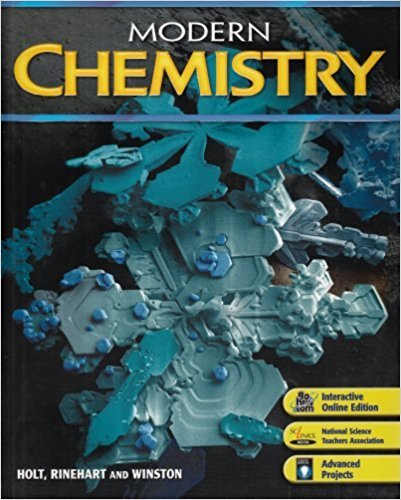Modern Chemistry - 1 Edition - Chapter 5 - Problem 20
Register Now

Join StudySoup

Get Full Access to Modern Chemistry - 1 Edition - Chapter 5 - Problem 209780030735462

# Without looking at the periodic table, write the expected outer electron configuration

Modern Chemistry | 1st Edition

Problem 20

Without looking at the periodic table, write the expected outer electron configuration for each of the following elements. (Hint: See Sample C.) a. Group 7, fourth period b. Group 3, fifth period c. Group 12, sixth period

Accepted Solution
Step-by-Step Solution:
Step 1 of 3

Friday, September 16, 2016 Week 3 Notes CHE 152 - Ions and the periodic table for main group elements: • - metals lose electrons (until they have the same number of electrons as the nearest noble gas) and become cations - nonmetals tend to gain electrons (until they have the same number of electrons as the nearest noble gas) and become anions • an atomic mass unit (amu) is exactly 1/12 the mass of a carbon-12 isotope • isotopic mass: the mass of a given isotope - the mass number =/= the mass on the periodic table (atomic mass) this is because the atomic mass is the weighted average of the isotopes of a • given element. found by taking the sum of iso

###### Chapter 5, Problem 20 is Solved

Step 2 of 3

Step 3 of 3

Unlock Textbook Solution